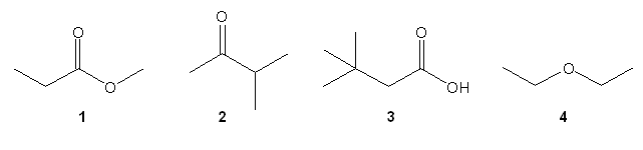Clutch Prep is now a part of Pearson
Ch. 15 - Analytical Techniques: IR, NMR, Mass SpectWorksheetSee all chapters

# NMR Integration

See all sections
Sections
Purpose of Analytical Techniques
Infrared Spectroscopy
Infrared Spectroscopy Table
IR Spect: Drawing Spectra
IR Spect: Extra Practice
NMR Spectroscopy
1H NMR: Number of Signals
1H NMR: Q-Test
1H NMR: E/Z Diastereoisomerism
H NMR Table
1H NMR: Spin-Splitting (N + 1) Rule
1H NMR: Spin-Splitting Simple Tree Diagrams
1H NMR: Spin-Splitting Complex Tree Diagrams
1H NMR: Spin-Splitting Patterns
NMR Integration
NMR Practice
Carbon NMR
Structure Determination without Mass Spect
Mass Spectrometry
Mass Spect: Fragmentation
Mass Spect: Isotopes

Concept #1: 1H NMR Integration

###### Expert Q&A

Ask unlimited questions and get expert help right away.

Transcript

Example #1: Draw Complete NMR Spectrum

###### Expert Q&A

Ask unlimited questions and get expert help right away.

Transcript

Alright guys, let's start off with the order of the protons and what we would see is that the most deshielded should be HC because HC is the closest to my electronegative atom and the most shielded should be HA. So, my order should have been HA here, HB here and HC here, because I'm assuming that HA is going to be the one closest to 0 and HC is going to be the one further down the field because the most deshielded, okay? That takes care of chemical shifts. Now, in terms of splitting, we have to use N plus 1. So, for HA I would say that N is equal to two, right? So, it's got two here. So, that means that it should be a triplet. So, I should draw a triplet for HA like that, okay? Now, for HB, what I see is a little bit more complicated, HB is getting split by three on one side and by one on the other, since we're assuming N plus 1 we can just add that all together and that's going to be, give it a second, that's going to be N equal to four, which means that we would get a quintet. So, remember that a quintet would be I believe the 1-4-6-4-1 pattern as predicted by Pascal's triangle. So, then we would try to draw that as best you can. So, 1-4-6-4-, okay? if you're not perfect it's not a big deal but we'll try do it as best as we can then finally HC. So, HC is being split by how many protons? Well, two on this side and two on this side. So, once again for HC N is equal to 4. So, we're going to get another quintet. So, let's do the same exact thing. So, it's not as nice, okay? So, there we go, we have our triple quintet and quintet and now all we need is integrations, okay? So, HA has how many hydrogen's that are of that type? six, this should be an integration of 6H why? Because we've got these three here, but I've also got these three here, remember, there was symmetry otherwise it would have had their own peak but they're symmetry. So, they're both the same. So, should be six hydrogens are type 6a, 6b how many type of that are there? Well, that's going to be 4H because I have two and two and then finally HC how many are there? just one H, okay? So, that is our fill out problem and now notice that by making these boxes and putting a kind of an order it made it easier to fill out but this is all the basics of drawing your own NMR spectrum. So, really from here. Now, after this practice problem I should be able to give you a blank NMR spectrum and you should be able to draw pretty much every single peak, every single signal, all the different splits, maybe even take a stab at the integration just from all this information, okay? Now, by the way, if you were to represent this as a ratio, the ratio would have been 1:4:6, okay? So, it's that easy. Alright, now if you do get a ratio that has multiples of numbers, let's say that, I'm just going to give a crazy example, let's say this molecule has been twice as big, so it was actually 2h it was actually 8h and it was actually 12 H, let's just say, this is just theoretical, okay? if that was the ratio then it could still be expressed like this, it could still be source with this because you could simplify all the numbers, you could divide them all by two and then you'd say? Well, there's more hydrogens but they're still in a 1:4:6 ratio. So, I'm just trying to show you that your ratios don't always add up the amount of protons you have, what they do tell you is the relative amount of each type of proton that you have, alright? so hope that made sense, let's go ahead and move on to the next topic.

Practice: PRACTICE: Which of the following molecules gives a 1H NMR spectrum consisting of three peaks with integral ratio of 3:1:6?###### Expert Q&A

Ask unlimited questions and get expert help right away.